[BACK]Computer Modeling in Engineering & SciencesDOI: 10.32604/cmes.2021.016736

ARTICLE

Decision Making Algorithmic Approaches Based on Parameterization of Neutrosophic Set under Hypersoft Set Environment with Fuzzy, Intuitionistic Fuzzy and Neutrosophic Settings

1Department of Mathematics, University of Management and Technology, Lahore, 54000, Pakistan
2Department of Mathematics, College of Science and Arts, Qassim University, Al-Rass, 51921, Saudi Arabia
3Department of Operations Research, Faculty of Graduate Studies for Statistical Research, Cairo University, Giza, 12613, Egypt
4Department of Mathematics, College of Science and Arts, Qassim University, Al-Badaya, 51951, Saudi Arabia
*Corresponding Author: Atiqe Ur Rahman. Email: aurkhb@gmail.com
Received: 23 March 2021; Accepted: 07 May 2021

Abstract: Hypersoft set is an extension of soft set as it further partitions each attribute into its corresponding attribute-valued set. This structure is more flexible and useful as it addresses the limitation of soft set for dealing with the scenarios having disjoint attribute-valued sets corresponding to distinct attributes. The main purpose of this study is to make the existing literature regarding neutrosophic parameterized soft set in line with the need of multi-attribute approximate function. Firstly, we conceptualize the neutrosophic parameterized hypersoft sets under the settings of fuzzy set, intuitionistic fuzzy set and neutrosophic set along with some of their elementary properties and set theoretic operations. Secondly, we propose decision-making-based algorithms with the help of these theories. Moreover, illustrative examples are presented which depict the structural validity for successful application to the problems involving vagueness and uncertainties. Lastly, the generalization of the proposed structure is discussed.

Keywords: Neutrosophic set; hypersoft set; neutrosophic hypersoft set; parameterized soft set; parameterized hypersoft set

1  Introduction

Fuzzy sets theory (FST)  and intuitionistic fuzzy set theory (IFST)  are considered apt mathematical modes to tackle many intricate problems involving various uncertainties, in different mathematical disciplines. The former one emphasizes on the degree of true belongingness of a certain object from the initial sample space whereas the later one accentuates on degree of true membership and degree of non-membership with condition of their dependency on each other. These theories depict some kind of inadequacy regarding the provision of due status to degree of indeterminacy. Such impediment is addressed with the introduction of neutrosophic set theory (NST) [3,4] which not only considers the due status of degree of indeterminacy but also waives off the condition of dependency. This theory is more flexible and appropriate to deal with uncertainty and vagueness. NST has attracted the keen concentration of many researchers  to further utilization in statistics, topological spaces as well as in the development of certain neutrosophic-like blended structures with other existing models for useful applications in decision making. Edalatpanah  studied a system of neutrosophic linear equations (SNLE) based on the embedding approach. He used (α,β,γ)-cut for transformation of SNLE into a crisp linear system. Kumar et al.  exhibited a novel linear programming approach for finding the neutrosophic shortest path problem (NSSPP) considering Gaussian valued neutrosophic number.

FST, IFST and NST have some kind of complexities which restrain them to solve problems involving uncertainty professionally. The reason for these hurdles is, possibly, the inadequacy of the parametrization tool. It demands a mathematical tool free of all such impediments to tackle such issues. This scantiness is resolved with the development of soft set theory (SST)  which is a new parameterized family of subsets of the universe of discourse. The researchers  studied and investigated some elementary properties, operations, laws and hybrids of SST with applications in decision making. The gluing concept of NST and SST, is studied in [35,36] to make the NST adequate with parameterized tool. In many real life situations, distinct attributes are further partitioned in disjoint attribute-valued sets but existing SST is insufficient for dealing with such kind of attribute-valued sets. Hypersoft set theory (HST)  is developed to make the SST in line with attribute-valued sets to tackle real life scenarios. HST is an extension of SST as it transforms the single argument approximate function into a multi-argument approximate function. Certain elementary properties, aggregation operations, laws, relations and functions of HST, are investigated by  for proper understanding and further utilization in different fields. The applications of HST in decision making is studied by  and the intermingling study of HST with complex sets, convex and concave sets is studied by [45,46]. Deli  characterized hybrid set structures under uncertainly parameterized hypersoft sets with theory and applications. Gayen et al.  analyzed some essential aspects of plithogenic hypersoft algebraic structures. They also investigated the notions and basic properties of plithogenic hypersoft subgroups, i.e., plithogenic fuzzy hypersoft subgroup, plithogenic intuitionistic fuzzy hypersoft subgroup, plithogenic neutrosophic hypersoft subgroup.

1.1 Motivation

In miscellany of real-life applications, the attributes are required to be further partitioned into attribute values for more vivid understanding. Hypersoft set as a generalization of soft set, accomplishes this limitation and accentuates the disjoint attribute-valued sets for distinct attributes. This generalization reveals that the hypersoft set with neutrosophic, intuitionistic, and fuzzy set theory will be very helpful to construct a connection between alternatives and attributes. It is interesting that the hypersoft theory can be applied on any decision-making problem without the limitations of the selection of the values by the decision-makers. This theory can successfully be applied to Multi-criteria decision making (MCDM), Multi-criteria group decision making (MCGDM), shortest path selection, employee selection, e-learning, graph theory, medical diagnosis, probability theory, topology, and many others. It is pertinent that the existing literature regarding soft set should be adequate with the existence and the consideration of attribute-valued sets, therefore, this study aims to develop novel theories of embedding structures of parameterized neutrosophic set and hypersoft set with the setting of fuzzy, intuitionistic fuzzy and neutrosophic sets through the extension of concept investigated in . Moreover, decision-making based algorithms are proposed for each setting to solve a real life problem relating to the purchase of most suitable and appropriate product with the help of some essential operations of these presented theories.

1.2 Organization of Paper

The rest of the paper is systemized as: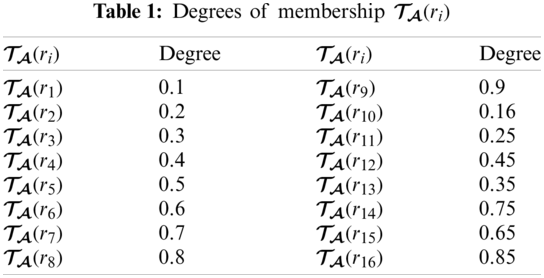2  Preliminaries

Here some basic terms are recalled from existing literature to support the proposed work. Throughout the paper, X, (X) and I will denote the universe of discourse, power set of X and closed unit interval respectively. In this work, algorithmic approaches are followed from decision making methods stated in .

Definition 2.1. 

A fuzzy set F defined as F={(â,AF(â))âX} such that AF:XI where AF(â) denotes the belonging value of âF.

Definition 2.2. 

An intuitionistic fuzzy set Y defined as Y={(b^,<AY(b^),BY(b^)>)b^X} such that AY:XI and BY:XI, where AY(b^) and BY(b^) denote the belonging value and not-belonging value of b^Y with condition of 0AY(b^)+BY(b^)1.

Definition 2.3. 

A neutrosophic set Z defined as Z={(ĉ,<AZ(ĉ),BZ(ĉ),CZ(ĉ)>)ĉX} such that AZ(ĉ),BZ(ĉ),CZ(ĉ):X(-0,1+), where AZ(ĉ),BZ(ĉ) and CZ(ĉ) denote the degrees of membership, indeterminacy and non-membership of ĉZ with condition of -0AZ(ĉ)+BZ(ĉ)+CZ(ĉ)3+.

Definition 2.4. 

A pair (FS,Λ) is called a soft set over X, where FS:Λ(X) and Λ be a subset of a set of attributes E.

For more detail on soft set, see .

Definition 2.5. 

The pair (W,G) is called a hypersoft set over X, where G is the cartesian product of n disjoint sets G1,G2,G3,,Gn having attribute values of n distinct attributes ĝ1,ĝ2,ĝ3,,ĝn respectively and W:G(X).

For more definitions and operations of hypersoft set, see .

3  Neutrosophic Parameterized Fuzzy Hypersoft Set (npfhs-Set) with Application

In this section, npfhs-set theory is conceptualized and a decision making application is discussed.

Definition 3.1. Let X={X1,X2,X3,,Xn} be a collection of disjoint attribute-valued sets corresponding to n distinct attributes α1,α2,α3,,αn, respectively. A npfhs-set ΨA over X is defined as ΨA={(<PA(g),QA(g),RA(g)>/g,ψA(g)):gG,ψA(g)F(X)} where

i)  F(X) is a collection of all fuzzy sets over X

ii)  G=X1×X2×X3××Xn

iii)  A is a neutrosophic set over G with PA,QA,RA:GI as membership function, indeterminacy function and nonmembership function of npfhs-set.

iv)  ψA(g) is a fuzzy set for all gG with ψA:GF(X) and is called approximate function of npfhs-set.

Note that collection of all npfhs-sets is represented by ΩNPFHS(X).

Definition 3.2. Let ΨAΩNPFHS(X). If ψA(g)=ϕ,PA(g)=0,QA(g)=1,RA(g)=1 for all gG, then ΨA is called A-empty npfhs-set, denoted by ΨΦA. If A=ϕ, then A-empty npfhs-set is called an empty npfhs-set, denoted by ΨΦ.

Definition 3.3. Let ΨAΩNPFHS(X). If ψA(g)=X,PA(g)=1,QA(g)=0,RA(g)=0 for all gG, then ΨA is called A-universal npfhs-set, denoted by ΨÃ. If A=G, then the A-universal npfhs-set is called universal npfhs-set, denoted by ΨG̃.

Example 3.1. Consider X={u1,u2,u3,u4,u5} and X={X1,X2,X3} with X1={x^11,x^12},

X2={x^21,x^22},X3={x^31}, then G=X1×X2×X3 G={(x^11,x^21,x^31),(x^11,x^22,x^31),(x^12,x^21,x^31),(x^12,x^22,x^31)}={g1,g2,g3,g4}.

Case 1.

If A1={<0.2,0.3,0.4>/g2,<0,1,1>/g3,<1,0,0>/g4} and

ψA1(g2)={0.4/u2,0.6/u4},ψA1(g3)=, and ψA1(g4)=X, thenΨA1={(<0.2,0.3,0.4>/g2,{0.4/u2,0.6/u4}),(<0,1,1>/g3,),(<1,0,0>/g4,X)}.

Case 2.

If A2={<0,1,1>/g2,<0,1,1>/g3},ψA2(g2)= and ψA2(g3)=, then ΨA2=ΨΦA2.

Case 3.

If A3= corresponding to all elements of G, then ΨA3=ΨΦ.

Case 4.

If A4={<1,0,0>/g1,<1,0,0>/g2},ψA4(g1)=X, and ψA4(g2)=X, then ΨA4=ΨA4̃.

Case 5.

If A5=X with respect to all elements of G, then ΨA5=ΨG̃.

Definition 3.4. Let ΨA1, ΨA2ΩNPFHS(X) then ΨA1 is an npfhs-subset of ΨA2, denoted by ΨA1̃fΨA2 if

PA1(g)PA2(g),QA1(g)QA2(g),RA1(g)RA2(g) and ψA1(g)fψA2(g)<transpanc/><d>:ltl:italic:gtl:for:ltl:/italic:gtl::ltl:italic:gtl:all:ltl:/italic:gtl:</d><transpanop/>gG.

Definition 3.5. Let ΨA1,ΨA2ΩNPFHS(X) then, ΨA1 and ΨA2 are npfhs-equal, represented as ΨA1=ΨA2, if and only if PA1(g)=PA2(g),QA1(g)=QA2(g),RA1(g)=RA2(g) and ψA1(g)=fψA2(g) for all gG.

Definition 3.6. Let ΨAΩNPFHS(X) then, complement of ΨA (i.e., ΨAc̃) is an npfhs-set given as PAc̃(g)=1-PA(g),QAc̃(g)=1-QA(g),RAc̃(g)=1-RA(g) and ψAc̃(g)=X\fψA(g).

Proposition 3.1. Let ΨAΩNPFHS(X) then,

1.    (ΨAc̃)c̃=ΨA.

2.    Ψϕc̃=ΨG̃.

Definition 3.7. Let ΨA1,ΨA2ΩNPFHS(X) then, union of ΨA1 and ΨA2, denoted by ΨA1̃fΨA2, is an npfhs-set defined by

i)  PA1̃A2(g)=max{PA1(x),PA2(g)},

ii)  QA1̃A2(g)=min{QA1(x),QA2(g)},

iii)  RA1̃A2(g)=min{RA1(x),RA2(g)},

iv)  ψA1̃A2(g)=ψA1(g)̃fψA2(g), for all gG.

Definition 3.8. Let ΨA1,ΨA2ΩNPFHS(X) then intersection of ΨA1 and ΨA2, denoted by ΨA1̃fΨA2, is an npfhs-set defined by

i)  PA1̃A2(g)=min{PA1(x),PA2(g)},

ii)  QA1̃A2(g)=max{QA1(x),QA2(g)},

iii)  RA1̃A2(g)=max{RA1(x),RA2(g)},

iv)  ψA1̃A2(g)=ψA1(g)̃fψA2(g), for all gG.

Remark 3.1. Let ΨAΩNPFHS(X). If ΨAfΨG̃, then ΨÃfΨAc̃fΨG̃ and ΨÃfΨAc̃fΨΦ

Proposition 3.2. Let ΨA1,ΨA2ΩNPFHS(X) D. Morgan laws are valid

1.    (ΨA1̃fΨA2)c̃=ΨA1c̃̃fΨA2c̃.

2.    (ΨA1̃fΨA2)c̃=ΨA1c̃̃fΨA2c̃.

Proof. For all gG,

(1).Since(PA1̃A2)c̃(g)=1-PA1̃A2(g)=1-max{PA1(g),PA2(g)}=min{1-PA1(g),1-PA2(g)}=min{PA1c̃(g),PA2c̃(g)}=PA1̃A2c̃(g)

also (QA1̃A2)c̃(g)=1-QA1̃A2(g)=1-min{QA1(g),QA2(g)}=max{1-QA1(g),1-QA2(g)}=max{QA1c̃(g),QA2c̃(g)}=QA1̃A2c̃(g) and (RA1̃A2)c̃(g)=1-RA1̃A2(g)=1-min{RA1(g),RA2(g)}=max{1-RA1(g),1-RA2(g)}=max{RA1c̃(g),RA2c̃(g)}=RA1̃A2c̃(g) and (ψA1̃A2)c̃(g)=X\fψA1̃A2(g)=X\f(ψA1(g)̃fψA2(g))=(X\fψA1(g))̃f(X\fψA2(g))=ψA1c̃(g)̃fψA2c̃(g)=ψA1̃A2c̃(g). similarly (2) can be proved easily.

Proposition 3.3. Let ΨA1, ΨA2, ΨA3ΩNPFHS(X) then

1.    ΨA1̃f(ΨA2̃fΨA3)=(ΨA1̃fΨA2)̃f(ΨA1̃fΨA3).

2.    ΨA1̃f(ΨA2̃fΨA3)=(ΨA1̃fΨA2)̃f(ΨA1̃fΨA3).

Proof. For all gG,

(1).SincePA1̃(A2̃A3)(g)=max{PA1(g),PA2̃A3(g)}=max{PA1(g),min{PA2(g),PA3(g)}}=min{max{PA1(g),PA2(g)},max{PA1(g),PA3(g)}}=min{PA1̃A2(g),PA1̃A3(g)}=P(A1̃A2)̃(A1̃A3)(g) and QA1̃(A2̃A3)(g)=min{QA1(g),QA2̃A3(g)}=min{QA1(g),max{QA2(g),QA3(g)}}=max{min{QA1(g),QA2(g)},min{QA1(g),QA3(g)}}=max{QA1̃A2(g),QA1̃A3(g)}=Q(A1̃A2)̃(A1̃A3)(g) and RA1̃(A2̃A3)(g)=min{RA1(g),RA2̃A3(g)}=min{RA1(g),max{RA2(g),RA3(g)}}=max{min{RA1(g),RA2(g)},min{RA1(g),RA3(g)}}=max{RA1̃A2(g),RA1̃A3(g)}=R(A1̃A2)̃(A1̃A3)(g) and ψA1̃(A2̃A3)(g)=ψA1(g)fψA2̃A3(g)=ψA1(g)f(ψA2(g)fψA3(g))=(ψA1(g)fψA2(g))f(ψA1(g)fψA3(g))=ψA1̃A2(g)fψA1ŨA3(g)=ψ(A1̃A2)̃(A1̃A3)(g)

In the same way, (2) can be proved.

Definition 3.9. Let ΨA1,ΨA2ΩNPFHS(X) then OR-operation of ΨA1 and ΨA2, denoted by ΨA1̃ΨA2, is an npfhs-set defined by

i)  PA1̃A2(g1,g2)=max{PA1(g1),PA2(g2)},

ii)  QA1̃A2(g1,g2)=min{QA1(g1),QA2(g2)},

iii)  RA1̃A2(g1,g2)=min{RA1(g1),RA2(g2)},

iv)  ψA1̃A2(g1,g2)=ψA1(g1)fψA2(g2), for all (g1,g2)A1×A2.

Definition 3.10. Let ΨA1,ΨA2ΩNPFHS(X) then AND-operation of ΨA1 and ΨA2, denoted by ΨA1̃ΨA2, is an npfhs-set defined by

i)  PA1̃A2(g1,g2)=min{PA1(g1),PA2(g2)},

ii)  QA1̃A2(g1,g2)=max{QA1(g1),QA2(g2)},

iii)  RA1̃A2(g1,g2)=max{RA1(g1),RA2(g2)},

iv)  ψA1̃A2(g1,g2)=ψA1(g1)fψA2(g2), for all (g1,g2)A1×A2.

Proposition 3.4. Let ΨA1,ΨA2,ΨA3ΩNPFHS(X) then

1.    ΨA1̃ΨΦ=ΨΦ.

2.    (ΨA1̃ΨA2)̃ΨA3=ΨA1̃(ΨA2̃ΨA3).

3.    (ΨA1̃ΨA2)̃ΨA3=ΨA1̃(ΨA2̃ΨA3).

3.1 Neutrosophic Decision Set of npfhs-Set

An algorithm is presented with the help of characterization of neutrosophic decision set on npfhs-set which based on decision making technique and is explained with example.

Definition 3.11. Let ΨAΩNPFHS(X) then a neutrosophic decision set of ΨA (i.e., ΨAD) is represented as

Algorithm 3.1. Once ΨAD has been established, it may be indispensable to select the best single substitute from the options. Therefore, decision can be set up with the help of following algorithm.

Step 1 Determine A={<TA(g),IA(g),FA(g)>/g:TA(g),IA(g),FA(g)I,gG},

Step 2 Find ψA(g)

Step 3 Construct ΨA over X,

Step 5 Choose the maximum of ζΨAD(u).

Example 3.2. Suppose that Mr. James Peter wants to buy a mobile tablet from a mobile market. There are eight kinds of tablets (options) which form the set of discourse X={T^1,T^2,T^3,T^4,T^5,T^6,T^7,T^8}. The best selection may be evaluated by observing the attributes, i.e., a1 = Storage (GB), a2 = Camera Resolution (mega pixels), a3 = Size (inches), a4 = RAM (GB), and a5 = Battery power (mAh). The attribute-valued sets corresponding to these attributes are:

A1={a11=64,a12=128}A2={a21=8,a22=16}A3={a31=10,a32=11}A4={a41=2,a42=4}A5={a51=5000} then =A1×A2×A3×A4×A5 ={r1,r2,r3,r4,,r16} where each ri,i=1,2,,16, is a 5-tuples element.

Step 1:

From Tabs. 13, we can construct A as

A={<0.1,0.2,0.3>/r1,<0.2,0.3,0.4>/r2,<0.3,0.4,0.5>/r3,<0.4,0.5,0.6>/r4,<0.5,0.6,0.7>/r5,<0.6,0.7,0.8>/r6,<0.7,0.8,0.9>/r7,<0.8,0.9,0.1>/r8,<0.9,0.1,0.2>/r9,<0.16,0.27,0.37>/r10,<0.25,0.35,0.45>/r11,<0.45,0.55,0.65>/r12,<0.35,0.45,0.55>/r13,<0.75,0.85,0.95>/r14,<0.65,0.75,0.85>/r15,<0.85,0.95,0.96>/r16}.

Step 2:

Tab. 4 presents ψA(ri) corresponding to each element of G.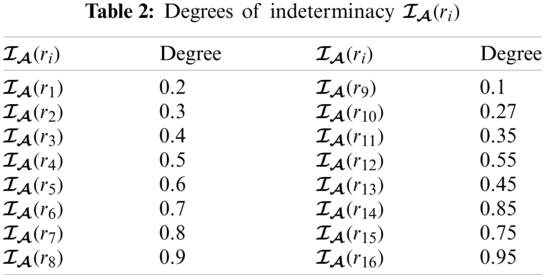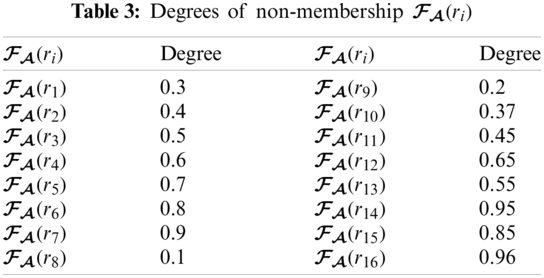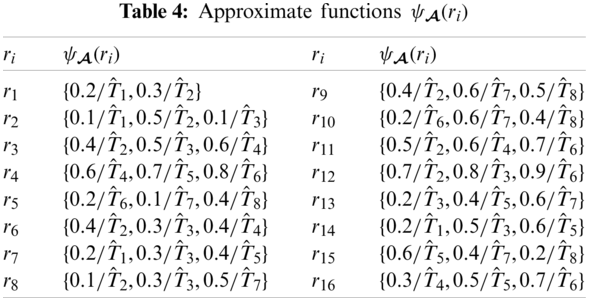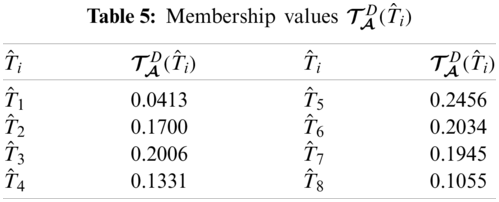Step 3:

With the help of Step 1 and Step 2, we can construct ΨA as

ΨA={(<0.1,0.2,0.3>/r1,{0.2/T^1,0.3/T^2}),(<0.2,0.3,0.4>/r2,{0.1/T^1,0.5/T^2,0.1T^3}),(<0.3,0.4,0.5>/r3,{0.4/T^2,0.5/T^3,0.6/T^4}),(<0.4,0.5,0.6>/r4,{0.6/T^4,0.7/T^5,0.8/T^6}),(<0.5,0.6,0.7>/r5,{0.2/T^6,0.1/T^7,0.4/T^8}),(<0.6,0.7,0.8>/r6,{0.4/T^2,0.3/T^3,0.4/T^4}),(<0.7,0.8,0.9>/r7,{0.2/T^1,0.3/T^3,0.4/T^5}),(<0.8,0.9,0.1>/r8,{0.1/T^2,0.3/T^3,0.5/T^7}),(<0.9,0.1,0.2>/r9,{0.4/T^2,0.6/T^7,0.5/T^8}),(<0.16,0.27,0.37>/r10,{0.2/T^6,0.6/T^7,0.4/T^8}),(<0.25,0.35,0.45>/r11,{0.5/T^2,0.6/T^4,0.7/T^6}),(<0.45,0.55,0.65>/r12,{0.7/T^2,0.8/T^3,0.9/T^6}),(<0.35,0.45,0.55>/r13,{0.2/T^3,0.4/T^5,0.6/T^7}),(<0.75,0.85,0.95>/r14,{0.2/T^1,0.5/T^3,0.6/T^5}),(<0.65,0.75,0.85>/r15,{0.6/T^5,0.4/T^7,0.2/T^8}),(<0.85,0.95,0.96>/r16,{0.3/T^4,0.5/T^5,0.7/T^6})}

Step 4:

From Tabs. 58, we can construct (ΨAD) as

The graphical representation of this decision system is presented in Fig. 1.

Step 5:

Since maximum of ζΨAD(T^i) is 0.2158 so the tablet T^7 is selected.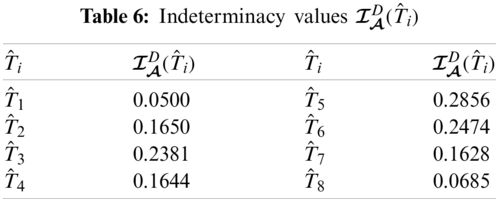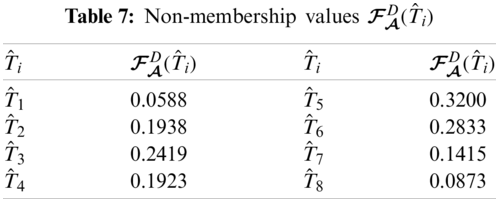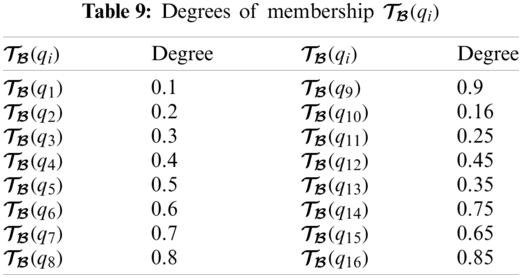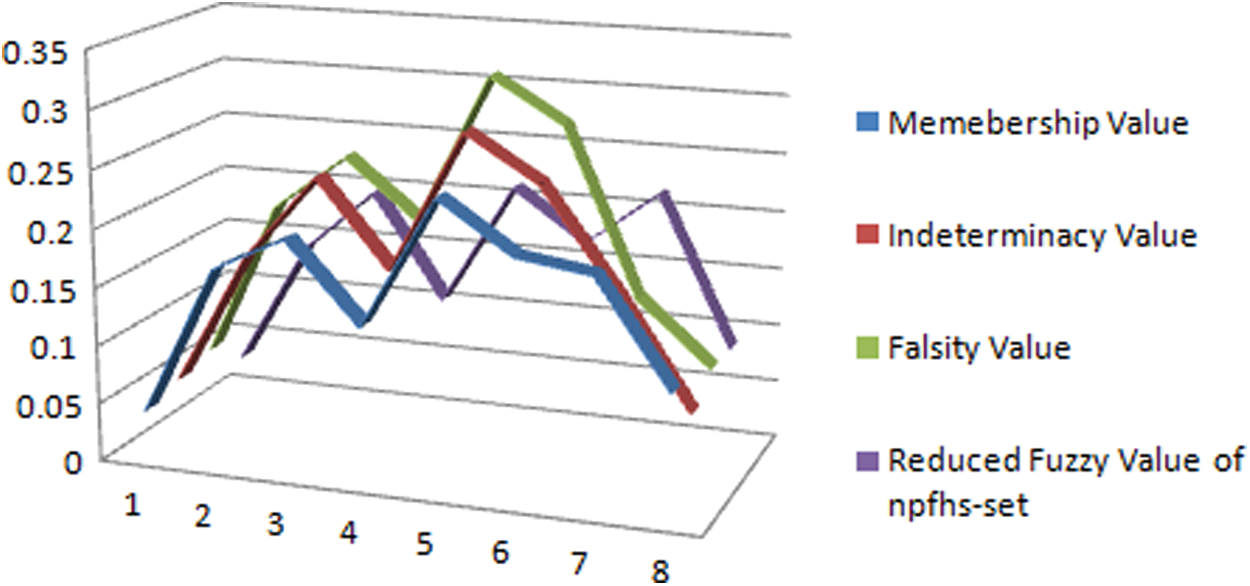Figure 1: Neutrosophic decision system on npfhs-set

4  Neutrosophic Parameterized Intuitionistic Fuzzy Hypersoft Set (npifhs-set) with Application

In this section, npifhs-set theory is developed and decision making based application is presented.

Definition 4.1. Let Y={Y1,Y2,Y3,,Yn} be a collection of disjoint attribute-valued sets corresponding to n distinct attributes α1,α2,α3,,αn, respectively. A npifhs-set ΨB over X is defined as ΨB={(<LB(g),MB(g),NB(g)>/g,ψB(g)):gG,ψA(g)IF(X)} where

i)  IF(U) is a collection of all intuitionistic fuzzy sets over X

ii)  G=Y1×Y2×Y3××Yn

iii)  B is a neutrosophic set over G with LB,MB,NB:GI as membership function, indeterminacy function and nonmembership function of npifhs-set.

iv)  ψB(g) is a fuzzy set for all gG with ψB:GIF(X) and is called approximate function of npifhs-set.

Note that collection of all npifhs-sets is represented by ΩNPIFHS(X).

Definition 4.2. Let ΨBΩNPIFHS(X). If ψB(g)=,LB(g)=0,MB(g)=1,NB(g)=1 for all gG, then ΨB is called B-empty npifhs-set, denoted by ΨΦB. If B=, then B-empty npifhs-set is called an empty npifhs-set, denoted by ΨΦ.

Definition 4.3. Let ΨBΩNPIFHS(X). If ψB(g)=X,LB(g)=1,MB(g)=0,NB(g)=0 for all gG, then ΨB is called B-universal npifhs-set, denoted by ΨB̃. If B=G, then the B-universal npifhs-set is called universal npifhs-set, denoted by ΨG̃.

Example 4.1. Consider X={u1,u2,u3,u4,u5} and Y={Y1,Y2,Y3} with

Y1={ŷ11,ŷ12},Y2={ŷ21,ŷ22},Y3={ŷ31}, thenG=Y1×Y2×Y3G={(ŷ11,ŷ21,ŷ31),(ŷ11,ŷ22,ŷ31),(ŷ12,ŷ21,ŷ31),(ŷ12,ŷ22,ŷ31)}={g1,g2,g3,g4}.

Case 1.

If B1={<0.2,0.3,0.4>/g2,<0,1,1>/g3,<1,0,0>/g4} and

ψB1(g2)={<0.2,0.4>/u2,<0.3,0.5>/u4},ψB1(g3)=ϕ, and ψB1(g4)=X, thenΨB1={(<0.2,0.3,0.4>/g2,{<0.2,0.4>/u2,<0.3,0.5>/u4}),(<0,1,1>/g3,ϕ),(<1,0,0>/g4,X)}.

Case 2.

If B2={<0,1,1>/g2,<0,1,1>/g3},ψB2(g2)=ϕ and ψB2(g3)=ϕ, then ΨB2=ΨΦB2.

Case 3.

If B3=ϕ corresponding to all elements of G, then ΨB3=ΨΦ.

Case 4.

If B4={<1,0,0>/g1,<1,0,0>/g2},ψB4(g1)=X, and ψB4(g2)=X, then ΨB4=ΨB4̃.

Case 5.

If B5=X with respect to all elements of G, then ΨB5=ΨG̃.

Definition 4.4. Let ΨB1, ΨB2ΩNPIFHS(X) then ΨB1 is an npifhs-subset of ΨB2, denoted by ΨB1̃ifΨB2 if

LB1(g)LB2(g),MB1(g)MB2(g),NB1(g)NB2(g) and ψB1(g)ifψB2(g) for all gG.

Definition 4.5. Let ΨB1,ΨB2ΩNPIFHS(X) then, ΨB1 and ΨB2 are npifhs-equal, represented as ΨB1=ΨB2, if and only if LB1(g)=LB2(g),MB1(g)=MB2(g),NB1(g)=NB2(g) and ψB1(g)=ψB2(g) for all gG.

Definition 4.6. Let ΨBΩNPIFHS(X) then, complement of ΨB (i.e., ΨBc̃) is an npifhs-set given as PBc̃(g)=1-LB(g),QBc̃(g)=1-MB(g),RBc̃(g)=1-NB(g) and ψBc̃(g)=X\ψB(g)

Proposition 4.1. Let ΨBΩNPIFHS(X) then,

1.    (ΨBc̃)c̃=ΨB.

2.    Ψϕc̃=ΨG̃.

Definition 4.7. Let ΨB1,ΨB2ΩNPIFHS(X) then, union of ΨB1 and ΨB2, denoted by ΨB1̃ifΨB2, is an npifhs-set defined by

i)  LB1̃B2(g)=max{LB1(x),LB2(g)},

ii)  MB1̃B2(g)=min{MB1(x),MB2(g)},

iii)  NB1̃B2(g)=min{NB1(x),NB2(g)},

iv)  ψB1̃B2(g)=ψB1(g)̃ifψB2(g), for all gG.

Definition 4.8. Let ΨB1,ΨB2ΩNPIFHS(X) then intersection of ΨB1 and ΨB2, denoted by ΨB1̃ifΨB2, is an npifhs-set defined by

i)  LB1̃B2(g)=min{LB1(x),LB2(g)},

ii)  MB1̃B2(g)=max{MB1(x),MB2(g)},

iii)  NB1̃B2(g)=max{NB1(x),NB2(g)},

iv)  ψB1̃B2(g)=ψB1(g)̃ifψB2(g), for all gG.

Remark 4.1. Let ΨBΩNPIFHS(X). If ΨBifΨG̃, then ΨB̃ifΨBc̃ifΨG̃ and ΨB̃if ΨBc̃ifΨΦ

Proposition 4.2. Let ΨB1,ΨB2ΩNPIFHS(X) then following D. Morgan laws are valid:

1.    (ΨB1̃ifΨB2)c̃=ΨB1c̃̃ifΨB2c̃.

2.    (ΨB1̃ifΨB2)c̃=ΨB1c̃̃ifΨB2c̃.

Proof. For all gG, (1).Since (LB1̃B2)c̃(g)=1-LB1̃B2(g)=1-max{LB1(g),LB2(g)}=min{1-LB1(g),1-LB2(g)}=min{PB1c̃(g),PB2c̃(g)}=PB1̃B2c̃(g) also (MB1̃B2)c̃(g)=1-MB1̃B2(g)=1-min{MB1(g),MB2(g)}=max{1-MB1(g),1-MB2(g)}=max{QB1c̃(g),QB2c̃(g)}=QB1̃B2c̃(g) and (NB1̃B2)c̃(g)=1-NB1̃B2(g)=1-min{NB1(g),NB2(g)}=max{1-NB1(g),1-NB2(g)}=max{RB1c̃(g),RB2c̃(g)}=RB1̃B2c̃(g) and (ψB1̃B2)c̃(g)=X\ifψB1̃B2(g)=X\if(ψB1(g)̃ifψB2(g))=(X\ifψB1(g))̃if(X\ifψB2(g))=ψB1c̃(g)̃ifψB2c̃(g)=ψB1̃B2c̃(g). similarly (2) can be proved easily.

Proposition 4.3. Let ΨB1,ΨB2,ΨB3ΩNPIFHS(X) then

1.    ΨB1̃if(ΨB2̃ifΨB3)=(ΨB1̃ifΨB2)̃if(ΨB1̃ifΨB3).

2.    ΨB1̃if(ΨB2̃ifΨB3)=(ΨB1̃ifΨB2)̃if(ΨB1̃ifΨB3).

Proof. For all gG,

(1).Since LB1̃(B2̃B3)(g)=max{LB1(g),LB2̃B3(g)}=max{LB1(g),min{LB2(g),LB3(g)}}=min{max{LB1(g),LB2(g)},max{LB1(g),LB3(g)}}=min{LB1̃B2(g),LB1̃B3(g)}=L(B1̃B2)̃(B1̃B3)(g) and MB1̃(B2̃B3)(g)=min{MB1(g),MB2̃B3(g)}=min{MB1(g),max{MB2(g),MB3(g)}}=max{min{MB1(g),MB2(g)},min{MB1(g),MB3(g)}}=max{MB1̃B2(g),MB1̃B3(g)}=M(B1̃B2)̃(B1̃B3)(g) and NB1̃(B2̃B3)(g)=min{NB1(g),NB2̃B3(g)}=min{NB1(g),max{NB2(g),NB3(g)}}=max{min{NB1(g),NB2(g)},min{NB1(g),NB3(g)}}=max{NB1̃B2(g),NB1̃B3(g)}=N(B1̃B2)̃(B1̃B3)(g) and ψB1̃(B2̃B3)(g)=ψB1(g)̃ifψB2̃B3(g)=ψB1(g)̃if(ψB2(g)̃ifψB3(g))=(ψB1(g)̃ifψB2(g))̃if(ψB1(g)̃ifψB3(g))=ψB1̃B2(g)̃ifψB1ŨB3(g)=ψ(B1̃B2)̃(B1̃B3)(g)

In the same way, (2) can be proved.

Definition 4.9. Let ΨB1,ΨB2ΩNPIFHS(X) then OR-operation of ΨB1 and ΨB2, denoted by ΨB1̃ΨB2, is an npifhs-set defined by

i)  LB1̃B2(g1,g2)=max{LB1(g1),LB2(g2)},

ii)  MB1̃B2(g1,g2)=min{MB1(g1),MB2(g2)},

iii)  NB1̃B2(g1,g2)=min{NB1(g1),NB2(g2)},

iv)  ψB1̃B2(g1,g2)=ψB1(g1)ψB2(g2), for all (g1,g2)B1×B2.

Definition 4.10. Let ΨB1,ΨB2ΩNPIFHS(X) then AND-operation of ΨB1 and ΨB2, denoted by ΨB1̃ΨB2, is an npifhs-set defined by

i)  LB1̃B2(g1,g2)=min{LB1(g1),LB2(g2)},

ii)  MB1̃B2(g1,g2)=max{MB1(g1),MB2(g2)},

iii)  NB1̃B2(g1,g2)=max{NB1(g1),NB2(g2)},

iv)  ψB1̃B2(g1,g2)=ψB1(g1)ψB2(g2), for all (g1,g2)B1×B2.

Proposition 4.4. Let ΨB1,ΨB2,ΨB3ΩNPIFHS(X) then

1.    ΨB1̃ΨΦ=ΨΦ.

2.    (ΨB1̃ΨB2)̃ΨB3=ΨB1̃(ΨB2̃ΨB3).

3.    (ΨB1̃ΨB2)̃ΨB3=ΨB1̃(ΨB2̃ΨB3).

4.1 Neutrosophic Decision Set of npifhs-Set

Here an algorithm is presented with the help of characterization of neutrosophic decision set on npifhs-set which based on decision making technique and is explained with example.

Definition 4.11. Let ΨBΩNPIFHS(X) then a neutrosophic decision set of ΨB (i.e., ΨBD) is represented as

ΨBD={<TBD(u),IBD(u),FBD(u)>/u:uX} where TBD,IBD,FBD:XI and TBD(u)=1|X|vS(B)TB(v)ΓψB(v)(u)IBD(u)=1|X|vS(B)IB(v)ΓψB(v)(u)FBD(u)=1|X|vS(B)FB(v)ΓψB(v)(u) where || denotes set cardinality with ΓψB(v)(u)={|TψB(u)-FψB(u)|;uΓψB(v)0;uΓψB(v)

Definition 4.12. If ΨBΩNPIFHS(X) with neutrosophic decision set ΨBD then reduced fuzzy set of ΨBD is a fuzzy set represented as (ΨBD)={ζΨBD(u)/u:uX} where ζΨBD:XI with ζΨBD(u)=TBD(u)+IBD(u)-FBD(u)

4.2 Proposed Algorithm

Once ΨBD has been established, it may be indispensable to select the best single substitute from the options. Therefore, decision can be set up with the help of following algorithm:

Step 1 Determine B={<TB(g),IB(g),FB(g)>/g:TB(g),IB(g),FB(g)I,gG},

Step 2 Find ψB(g)

Step 3 Construct ΨB over X,

Step 4 Compute ΨBD,

Step 5 Choose the maximum of ζΨBD(u).

Example 4.2. Suppose that Mrs. Andrew wants to buy a washing machine from market. There are eight kinds of washing machines (options) which form the set of discourse X={Ŵ1,Ŵ2,Ŵ3,Ŵ4,Ŵ5,Ŵ6,Ŵ7,Ŵ8}. The best selection may be evaluated by observing the attributes i.e., b1 = Company, b2 = Power in Watts, b3 = Voltage, b4 = Capacity in kg, and b5 = Color. The attribute-valued sets corresponding to these attributes are:

B1={b11=National,b12=Hier}B2={b21=400,b22=500}B3={b31=220,b32=240}B4={b41=7,b42=10}B5={b51=White} then =B1×B2×B3×B4×B5 ={q1,q2,q3,q4,,q16} where each qi,i=1,2,,16, is a 5-tuples element.

Step 1:

From Tabs. 911, we can construct B as

B={<0.1,0.2,0.3>/q1,<0.2,0.3,0.4>/q2,<0.3,0.4,0.5>/q3,<0.4,0.5,0.6>/q4,<0.5,0.6,0.7>/q5,<0.6,0.7,0.8>/q6,<0.7,0.8,0.9>/q7,<0.8,0.9,0.1>/q8,<0.9,0.1,0.2>/q9,<0.16,0.27,0.37>/q10,<0.25,0.35,0.45>/q11,<0.45,0.55,0.65>/q12,<0.35,0.45,0.55>/q13,<0.75,0.85,0.95>/q14,<0.65,0.75,0.85>/q15,<0.85,0.95,0.96>/q16}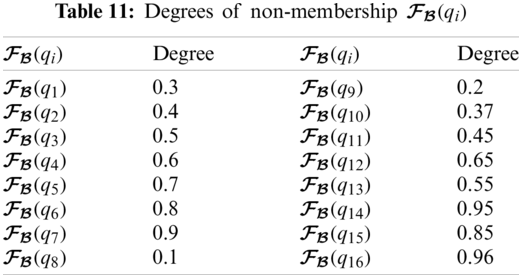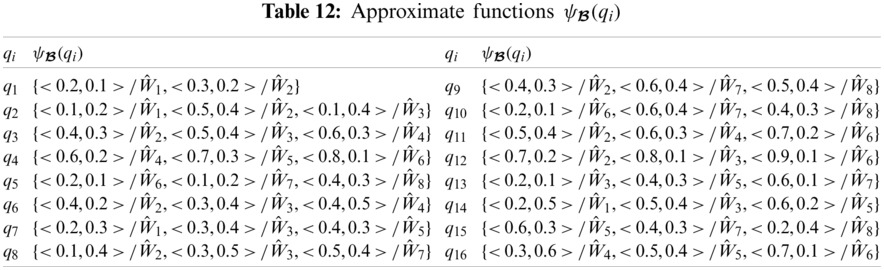Step 2:

Tab. 12 presents ψB(qi) corresponding to each element of G.Step 3: With the help of Step 1 and Step 2, we can construct ΨB as performed in step of Section 3.

Step 4:

From Tabs. 1316, we can construct (ΨBD) as

(ΨBD)={0.0331/Ŵ1,0.1100/Ŵ2,0.1019/Ŵ3,0.0659/Ŵ4,0.0855/Ŵ5,0.1394/Ŵ6,0.0690/Ŵ7,0.0296/Ŵ8}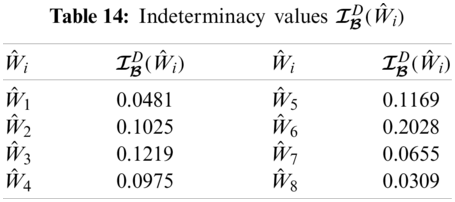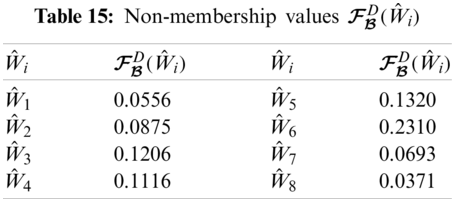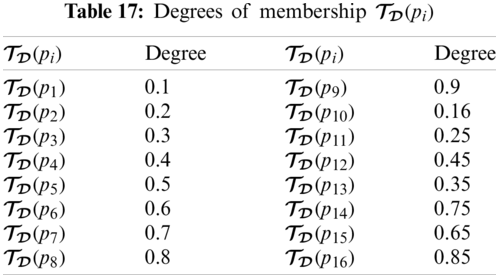The graphical representation of this decision system is presented in Fig. 2.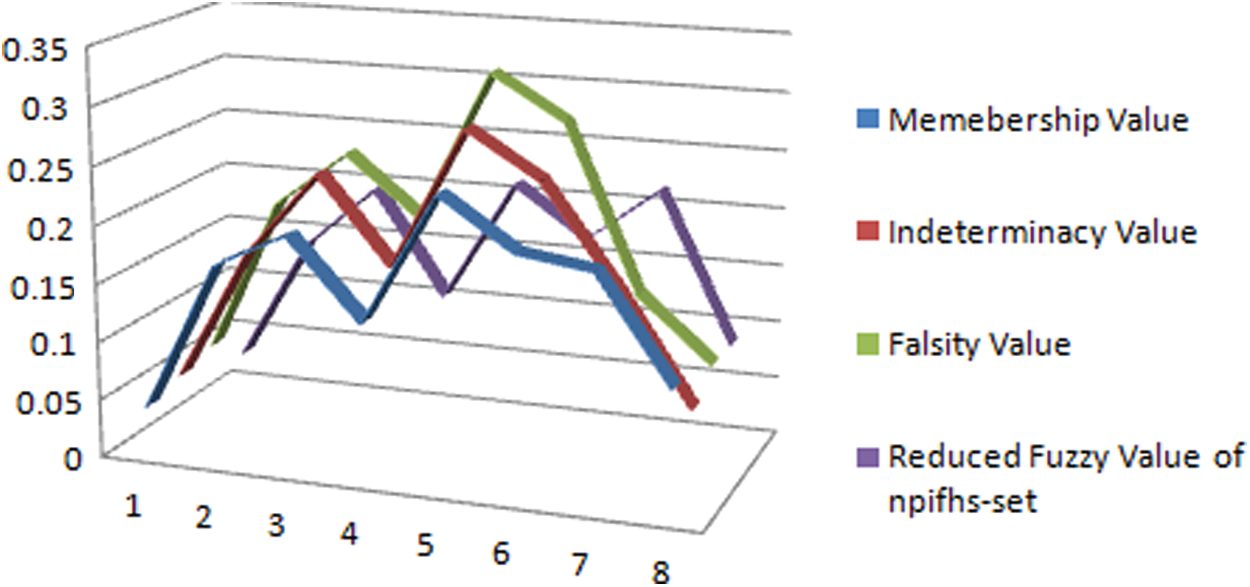Figure 2: Neutrosophic decision system on npifhs-set

Step 5:

Since maximum of ζΨBD(Ŵi) is 0.5313 so the washing machine Ŵ3 is selected.

5  Neutrosophic Parameterized Neutrosophic Hypersoft Set (npnhs-Set) with Application

In this section, neutrosophic parameterized hypersoft set is conceptualized and some of its fundamentals are discussed.

Definition 5.1. Let Z={Z1,Z2,Z3,,Zn} be a collection of disjoint attribute-valued sets corresponding to n distinct attributes α1,α2,α3,,αn, respectively. A npnhs-set ΨD over X is defined as ΨD={(<AD(g),BD(g),CD(g)>/g,ψD(g)):gG,ψD(g)N(X)} where

i)  N(X) is a collection of all neutrosophic sets over X

ii)  G=Z1×Z2×Z3××Zn

iii)  D is a neutrosophic set over G with AD,BD,CD:GI as membership function, indeterminacy function and nonmembership function of npnhs-set.

iv)  ψD(g) is a neutrosophic set for all gG with ψD:GN(X) and is called approximate function of npnhs-set.

Note that collection of all npnhs-sets is represented by ΩNPNHS(X).

Definition 5.2. Let ΨDΩNPNHS(X). If ψD(g)=,AD(g)=0,BD(g)=1,CD(g)=1 for all gG, then ΨD is called D-empty npnhs-set, denoted by ΨΦD. If D=, then D-empty npnhs-set is called an empty npnhs-set, denoted by ΨΦ.

Definition 5.3. Let ΨDΩNPNHS(X). If ψD(g)=X,AD(g)=1,BD(g)=0,CD(g)=0 for all gG, then ΨD is called D-universal npnhs-set, denoted by ΨD̃. If D=G, then the D-universal npnhs-set is called universal npnhs-set, denoted by ΨG̃.

Example 5.1. Consider X={u1,u2,u3,u4,u5} and Z={Z1,Z2,Z3} with Z1={11,12}, Z2={21,22},Z3={31}, then

G=Z1×Z2×Z3G={(11,21,31),(11,22,31),(12,21,31),(12,22,31)}={g1,g2,g3,g4}.

Case 1.

If D1={<0.2,0.3,0.4>/g2,<0,1,1>/g3,<1,0,0>/g4} and

ψD1(g2)={<0.2,0.4,0.6>/u2,<0.3,0.5,0.7>/u4},ψD1(g3)=, and ψD1(g4)=X, thenΨD1={(<0.2,0.3,0.4>/g2,{<0.2,0.4,0.6>/u2,0.3,0.5,0.7>/u4}),(<0,1,1>/g3,),(<1,0,0>/g4,X)}.

Case 2.

If D2={<0,1,1>/g2,<0,1,1>/g3},ψD2(g2)= and ψD2(g3)=, then ΨD2=ΨΦD2.

Case 3.

If D3= corresponding to all elements of G, then ΨD3=ΨΦ.

Case 4.

If D4={<1,0,0>/g1,<1,0,0>/g2},ψD4(g1)=X, and ψD4(g2)=X, then ΨD4=ΨD4̃.

Case 5.

If D5=X with respect to all elements of G, then ΨD5=ΨG̃.

Definition 5.4. Let ΨD1, ΨD2ΩNPNHS(X) then ΨD1 is an npnhs-subset of ΨD2, denoted by ΨD1̃ΨD2 if AD1(g)AD2(g),BD1(g)BD2(g),CD1(g)CD2(g) and ψD1(g)nψD2(g) for all gG.

Proposition 5.1. Let ΨD1,ΨD2,ΨD3ΩNPNHS(X) then

1.    ΨD1̃ΨG̃.

2.    ΨΦ̃ΨD1.

3.    ΨD1̃ΨD1.

4.    if ΨD1̃ΨD2 and ΨD2̃ΨD3 then ΨD1̃ΨD3.

Definition 5.5. Let ΨD1,ΨD2ΩNPNHS(X) then, ΨD1 and ΨD2 are npnhs-equal, represented as ΨD1=ΨD2, if and only if AD1(g)=AD2(g),BD1(g)=BD2(g),CD1(g)=CD2(g) and ψD1(g)=nψD2(g) for all gG.

Proposition 5.2. Let ΨD1,ΨD2,ΨD3ΩNPNHS(X) then,

1.    if ΨD1=ΨD2 and ΨD2=ΨD3 then ΨD1=ΨD3.

2.    if ΨD1̃ΨD2 and ΨD2̃ΨD1ΨD1=ΨD2.

Definition 5.6. Let ΨDΩNPNHS(X) then, complement of ΨD (i.e., ΨDc̃) is a npnhs-set given as PDc̃(g)=1-AD(g),QDc̃(g)=1-BD(g),RDc̃(g)=1-CD(g) and ψDc̃(g)=X\nψD(g).

Proposition 5.3. Let ΨDΩNPNHS(X) then,

1.    (ΨDc̃)c̃=ΨD.

2.    Ψϕc̃=ΨG̃.

Definition 5.7. Let ΨD1,ΨD2ΩNPNHS(X) then, union of ΨD1 and ΨD2, denoted by ΨD1̃ΨD2, is an npnhs-set defined by

ii)  BD1̃D2(g)=min{BD1(x),BD2(g)},

iii)  CD1̃D2(g)=min{CD1(x),CD2(g)},

iv)  ψD1̃D2(g)=ψD1(g)nψD2(g), for all gG.

Proposition 5.4. Let ΨD1,ΨD2,ΨD3ΩNPNHS(X) then,

1.    ΨD1̃ΨD1=ΨD1,

2.    ΨD1̃ΨΦ=ΨD1,

3.    ΨD1̃ΨG̃=ΨG̃,

4.    ΨD1̃ΨD2=ΨD2̃ΨD1,

5.    (ΨD1̃ΨD2)̃ΨD3=ΨD1̃(ΨD2̃ΨD3).

Definition 5.8. Let ΨD1,ΨD2ΩNPNHS(X) then intersection of ΨD1 and ΨD2, denoted by ΨD1̃ΨD2, is an npnhs-set defined by

ii)  BD1̃D2(g)=max{BD1(x),BD2(g)},

iii)  CD1̃D2(g)=max{CD1(x),CD2(g)},

iv)  ψD1̃D2(g)=ψD1(g)nψD2(g), for all gG.

Proposition 5.5. Let ΨD1,ΨD2,ΨD3ΩNPNHS(X) then

1.    ΨD1̃ΨD1=ΨD1.

2.    ΨD1̃ΨΦ=ΨΦ.

3.    ΨD1̃ΨG̃=ΨD1̃.

4.    ΨD1̃ΨD2=ΨD2̃ΨD1.

5.    (ΨD1̃ΨD2)̃ΨΨD3=ΨD1̃(ΨD2̃ΨΨD3).

Note: It is pertinent to mention here that Propositions 5.1, 5.2, 5.4 and 5.5 are also valid for elements of ΩNPFHS(X) and ΩNPIFHS(X).

Remark 5.1. Let ΨDΩNPNHS(X). If ΨDΨG̃, then ΨD̃ΨDc̃ΨG̃ and ΨD̃ΨDc̃ΨΦ

Proposition 5.6. Let ΨD1,ΨD2ΩNPNHS(X) then following D. Morgan laws are valid:

1.    (ΨD1̃ΨD2)c̃=ΨD1c̃̃ΨD2c̃.

2.    (ΨD1̃ΨD2)c̃=ΨD1c̃̃ΨD2c̃.

Proposition 5.7. Let ΨD1,ΨD2,ΨD3ΩNPNHS(X) then

1.    ΨD1̃(ΨD2̃ΨD3)=(ΨD1̃ΨD2)̃(ΨD1̃ΨD3).

2.    ΨD1̃(ΨD2̃ΨD3)=(ΨD1̃ΨD2)̃(ΨD1̃ΨD3).

Proof. For all gG,

In the same way, (2) can be proved.

Definition 5.9. Let ΨD1,ΨD2ΩNPNHS(X) then OR-operation of ΨD1 and ΨD2, denoted by ΨD1̃ΨD2, is an npnhs-set defined by

ii)  BD1̃D2(g1,g2)=min{BD1(g1),BD2(g2)},

iii)  CD1̃D2(g1,g2)=min{CD1(g1),CD2(g2)},

iv)  ψD1̃D2(g1,g2)=ψD1(g1)ψD2(g2), for all (g1,g2)D1×D2.

Definition 5.10. Let ΨD1,ΨD2ΩNPNHS(X) then AND-operation of ΨD1 and ΨD2, denoted by ΨD1̃ΨD2, is an npnhs-set defined by

ii)  BD1̃D2(g1,g2)=max{BD1(g1),BD2(g2)},

iii)  CD1̃D2(g1,g2)=max{CD1(g1),CD2(g2)},

iv)  ψD1̃D2(g1,g2)=ψD1(g1)ψD2(g2), for all (g1,g2)D1×D2.

Proposition 5.8. Let ΨD1,ΨD2,ΨD3ΩNPNHS(X) then

1.    ΨD1̃ΨΦ=ΨΦ.

2.    (ΨD1̃ΨD2)̃ΨD3=ΨD1̃(ΨD2̃ΨD3).

3.    (ΨD1̃ΨD2)̃ΨD3=ΨD1̃(ΨD2̃ΨD3).

5.1 Neutrosophic Decision Set of npnhs-Set

Here an algorithm is presented with the help of characterization of neutrosophic decision set on npnhs-set which based on decision making technique and is explained with example.

Definition 5.11. Let ΨDΩNPNHS(X) then a neutrosophic decision set of ΨD (i.e., ΨDD) is represented as

ΨDD={<TDD(u),IDD(u),FDD(u)>/u:uX} where TDD,IDD,FDD:XI and TDD(u)=1|X|vS(D)TD(v)ΓψD(v)(u)IDD(u)=1|X|vS(D)ID(v)ΓψD(v)(u)FDD(u)=1|X|vS(D)FD(v)ΓψD(v)(u) where || denotes set cardinality with ΓψD(v)(u)={|TψD(u)+IψD(u)-FψD(u)|;uΓψD(v)0;uΓψD(v)

Definition 5.12. If ΨDΩNPNHS(X) with neutrosophic decision set ΨDD then reduced fuzzy set of ΨDD is a fuzzy set represented as (ΨDD)={ζΨDD(u)/u:uX} where ζΨDD:XI with ζΨDD(u)=TDD(u)+IDD(u)-FDD(u).

5.2 Proposed Algorithm

Once ΨDD has been established, it may be indispensable to select the best single substitute from the options. Therefore, decision can be set up with the help of following algorithm:

Step 1 Determine D={<TD(g),ID(g),FD(g)>/g:TD(g),ID(g),FD(g)I,gG},

Step 2 Find ψD(g)

Step 3 Construct ΨD over X,

Step 4 Compute ΨDD,

Step 5 Choose the maximum of ζΨDD(u).

Hand sanitizer is a liquid or gel mostly used to diminish infectious agents on the hands. According to the World Health Organization (WHO), in current epidemic circumstances of COVID-19, high-quality sanitation and physical distancing are the best ways to protect ourselves and everyone around us from this virus. This virus spreads by touching an ailing person. We cannot detach ourselves totally being cautious from this virus. So, high-quality sanitation can be the ultimate blockade between us and the virus. Alcohol-based hand sanitizers are recommended by WHO to remove the novel corona virus. Alcohol-based hand sanitizers avert the proteins of germs including bacteria and some viruses from functioning normally. Demand of a hand sanitizer has been increased terrifically in such serious condition of COVID-19. Therefore, it is tricky to have good and effectual hand sanitizers in local markets. Low quality hand sanitizers have also been introduced due to its increasing demand. The core motivation of this application is to select an effectual sanitizer to alleviate the spread of corona virus by applying the NPNHS-set theory.

Example 5.2. Suppose that Mr. William wants to purchase an effective hand sanitizer from the local market. There are eight kinds of Hand Sanitizer (options) which form the set of discourse

X={H1,H2,H3,H4,H5,H6,H7,H8}.

The best selection may be evaluated by observing the attributes i.e., k1 = Manufacturer, k2 = Quantity of Ethanol (percentage), k3 = Quantity of Distilled Water (percentage), k4 = Quantity of Glycerol (percentage), and k5 = Quantity of Hydrogen peroxide (percentage). The attribute-valued sets corresponding to these attributes are:

K1={k11=ProcterandGamble,k12=Unilever}K2={k21=75.15,k22=80}K3={k31=23.425,k32=18.425}K4={k41=1.30,k42=1.45}K5={k51=0.125} then =K1×K2×K3×K4×K5 ={p1,p2,p3,p4,,p16} where each pi,i=1,2,,16,is a 5-tuples element.

Step 1:

From Tabs. 1719, we can construct D as

D={<0.1,0.2,0.3>/p1,<0.2,0.3,0.4>/p2,<0.3,0.4,0.5>/p3,<0.4,0.5,0.6>/p4,<0.5,0.6,0.7>/p5,<0.6,0.7,0.8>/p6,<0.7,0.8,0.9>/p7,<0.8,0.9,0.1>/p8,<0.9,0.1,0.2>/p9,<0.16,0.27,0.37>/p10,<0.25,0.35,0.45>/p11,<0.45,0.55,0.65>/p12,<0.35,0.45,0.55>/p13,<0.75,0.85,0.95>/p14,<0.65,0.75,0.85>/p15,<0.85,0.95,0.96>/p16}.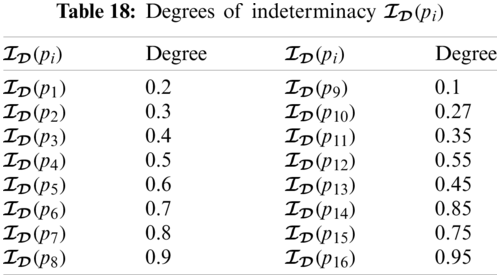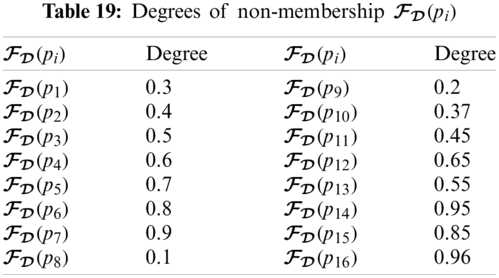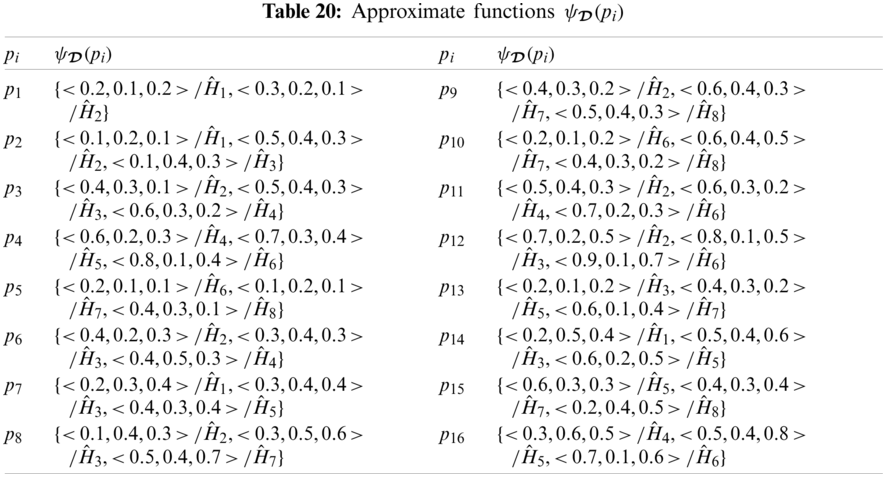Step 2:

Tab. 20 presents ψD(pi) corresponding to each element of G.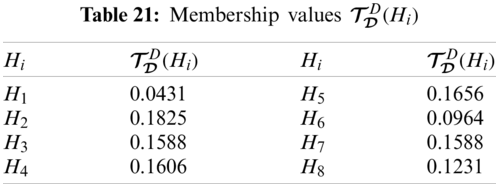Step 3:

ΨD can be constructed with the help of Step 1 and Step 2 same as done in Step 3 of Section 3.

Step 4:

From Tabs. 2124, we can construct (ΨDD) as

(ΨDD)={0.0344/H1,0.1600/H2,0.1500/H3,0.1289/H4,0.1367/H5,0.0749/H6,0.1538/H7,0.1006/H8}.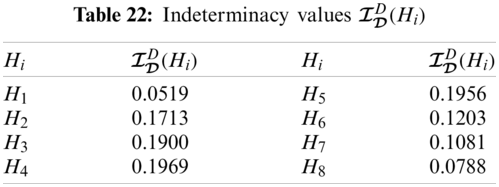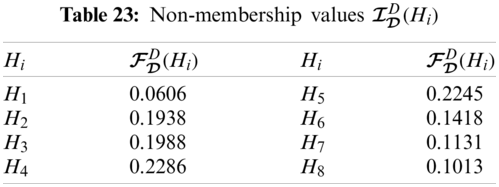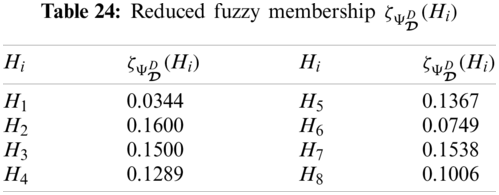The graphical representation of this decision system is presented in Fig. 3.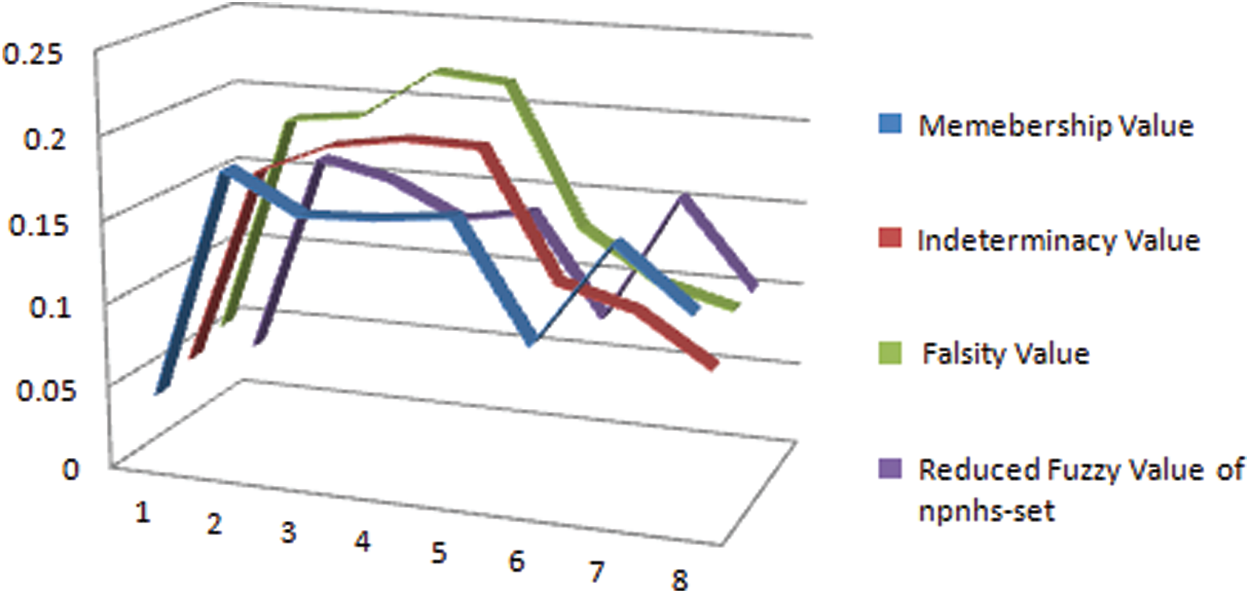Figure 3: Neutrosophic decision system on npnhs-set

Step 5:

Since maximum of ζΨDD(Hi) is 0.1600 so the Hand Sanitizer H2 is selected.

6  Discussion

The development and stability of any society depends on its justice system and the judges, lawyers and plaintiffs play a key role in its basic components. The lawyer prepares the writ petition at the request of the plaintiff but when filing the case in the Court of Justice, he/she is in a state of uncertainty for its success. This uncertain condition can be of fuzzy, intuitionistic fuzzy or even neutrosophic. And after the case is submitted, the judge concerned writes his/her decision in the light of the facts, but usually all facts have some kind of uncertainty. Such factual vagueness again may be of fuzzy, intuitionistic fuzzy or neutrosophic nature. So when initial stage (submission stage) and final stage (decisive stage) are neutrosophic valued and the process is executed with the help of parameterized data (collections of parametric values) then we say that we are tackling such problem with the help of neutrosophic parameterized neutrosophic hypersoft set (npnhs-set). Since decision makers always face some sort of uncertainties and any decision taken by ignoring uncertainty may have some extent of inclination. Indeterminacy and uncertainty are both interconnected. In this study, it has been shown (i.e., see Fig. 4) that how results are affected when indeterminacy is ignored or considered. Our proposed structure npnhs-set is very useful in dealing with many decisive systems and it is the generalization of:

i)  Neutrosophic Parameterized Intuitionistic Fuzzy Hypersoft Set (npifhs-set) if indeterminacy is ignored and remaining two are made interdependent within closed unit interval in approximate function of npnhs-set,

ii)  Neutrosophic Parameterized Fuzzy Hypersoft Set (npfhs-set) if indeterminacy and falsity are ignored and remaining be restricted within closed unit interval in approximate function of npnhs-set,

iii)  Neutrosophic Parameterized Hypersoft Set (nphs-set) if all uncertain components are ignored and approximate function of npnhs-set is a subset of universe of discourse,

iv)  Neutrosophic Parameterized Neutrosophic Soft Set (npns-set) if attribute-valued sets are replaced with only attributes in npnhs-set,

v)  Neutrosophic Parameterized Intuitionistic Fuzzy Soft Set (npifs-set) if attribute-valued sets are replaced with only attributes and indeterminacy is ignored and remaining two are made interdependent within closed unit interval in approximate function of npnhs-set,

vi)  Neutrosophic Parameterized Fuzzy Soft Set (npfs-set) if attribute-valued sets are replaced with only attributes and indeterminacy, falsity are ignored and remaining be restricted within closed unit interval in approximate function of npnhs-set,

vii)  Neutrosophic Parameterized Soft Set (nps-set) if attribute-valued sets are replaced with only attributes and all uncertain components are ignored with approximate function of npnhs-set as a subset of universe of discourse.

Fig. 5 presents the pictorial view of the generalization of the proposed structure.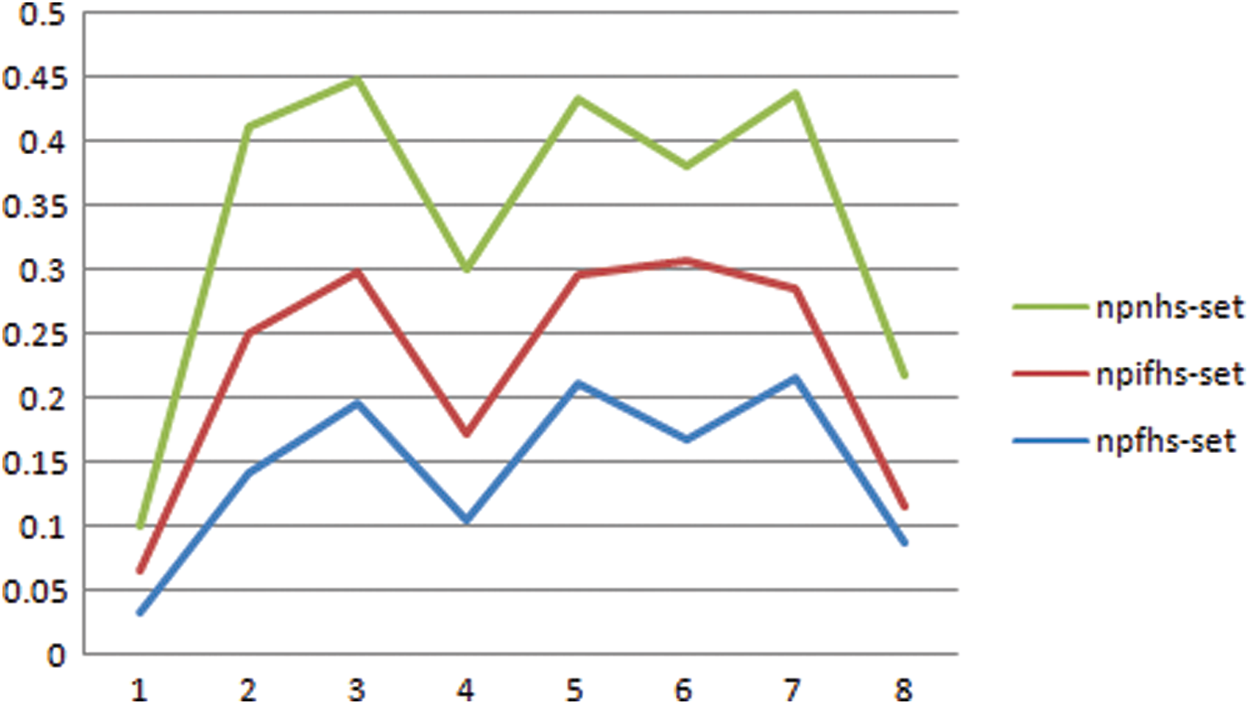Figure 4: Comparison of neutrosophic decision system on npfhs-set, npifhs-set and npnhs-set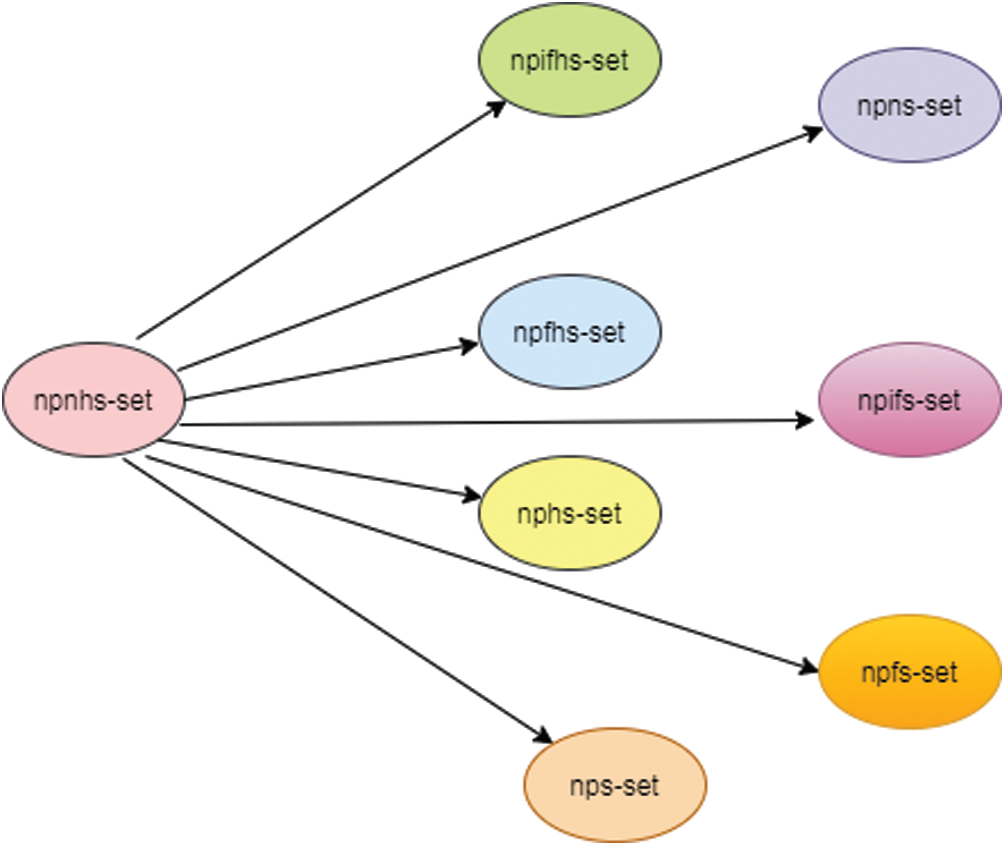Figure 5: Generalization of npnhs-set

7  Conclusion

In this study, neutrosophic parameterized hypersoft set is conceptualized for the environments of fuzzy set, intuitionistic fuzzy set and neutrosophic set along with some of their elementary properties and theoretic operations. Novel algorithms are proposed for decision making and are validated with the help of illustrative examples for appropriate purchasing of suitable products i.e., Mobile Tablet, Washing Machines and Hand Sanitizers, from the local market. Future work may include the extension of this work for:

•   The development of algebraic structures i.e., topological spaces, vector spaces, etc.,

•   The development of hybrid structures with fuzzy-like environments,

•   Dealing with decision making problems with multi-criteria decision making techniques,

•   Applying in medical diagnosis and optimization for agricultural yield,

•   Investigating and determining similarity, distance, dissimilarity measures and entropies between the proposed structures.

Funding Statement: The authors received no specific funding for this study.

Conflicts of Interest: The authors declare that they have no conflicts of interest to report regarding the present study.

References

1.  1.  Zadeh, L. (1965). Fuzzy sets. Information and Control, 8(3), 338–353. DOI 10.1016/S0019-9958(65)90241-X.
2.  2.  Atanassov, K. (1986). Intuitionistic fuzzy sets. Fuzzy Sets and Systems, 20(1), 87–96. DOI 10.1016/S0165-0114(86)80034-3.
3.  3.  Smarandache, F. (1998). Neutrosophy: Neutrosophic probability, set, and logic: Analytic synthesis and synthetic analysis. Rehoboth: American Research Press.
4.  4.  Smarandache, F. (1999). A Unifying field in logics. Neutrosophy: Neutrosophic probability, set and logic. Rehoboth: American Research Press.
5.  5.  Smarandache, F. (2005). Neutrosophic set, a generalization of intuitionistic fuzzy sets. International Journal of Pure and Applied Mathematics, 24(3), 287–297.
6.  6.  Smarandache, F. (2006). Neutrosophic set—A generalization of intuitionistic fuzzy set. International Conference on Granular Computing, pp. 38–42. Atlanta, Georgia, USA, IEEE.
7.  7.  Wang, H., Rogatko, A., Smarandache, F., Sunderraman, R. (2006). A Neutrosophic description logic. International Conference on Granular Computing, pp. 305–308. Atlanta, Georgia, USA, IEEE. DOI 10.1109/GRC.2006.1635801.
8.  8.  Smarandache, F. (2013). n-Valued refined neutrosophic logic and its applications in physics. Progress in Physics, 4, 143–146. DOI 10.5281/zenodo.49149.
9.  9.  Smarandache, F. (2016a). Operators on single valued neutrosophic sets, neutrosophic undersets, and neutrosophic offsets. Journal of Mathematics and Informatics, 5, 63–67. DOI 10.5281/zenodo.57412.
10. 10. Smarandache, F. (2016b). Neutrosophic overset, neutrosophic underset, neutrosophic offset, similarly for neutrosophicover-/under-/offlogic, probability, and statistic. Brussels: Pons Editions. DOI 10.5281/zenodo.57410.
11. 11. Broumi, S., Bakali, A., Talea, M., Smarandache, F., Karaaslan, F. (2016). Interval valued neutrosophic soft graphs. New Trends in Neutrosophic Theory and Applications, 2, 214–251. DOI 10.5281/zenodo.1410271.
12. 12. Alhabib, R., Ranna, M. M., Farah, H., Salama, A. A. (2018). Some neutrosophic probability distributions. Neutrosophic Sets and Systems, 22, 30–38. DOI 10.5281/zenodo.2160479.
13. 13. Pramanik, S., Dey, P. P., Smarandache, F. (2018). Correlation coefficient measures of interval bipolar neutrosophic sets for solving multi-attribute decision making problems. Neutrosophic Sets and Systems, 19, 70–79. DOI 10.5281/zenodo.1235151.
14. 14. Broumi, S., Smarandache, F. (2013). Intuitionistic neutrosophic soft set. Journal of Information and Computing Science, 8(2), 130–140. DOI 10.5281/zenodo.2861554.
15. 15. Broumi, S., Smarandache, F. (2013). More on intuitionistic neutrosophic soft set. Computer Science and Information Technology, 1(4), 257–268. DOI 10.13189/csit.2013.010404.
16. 16. Broumi, S. (2013). Generalized neutrosophic soft set. International Journal of Computer Science, Engineering and Information Technology, 3(2), 17–30. DOI 10.5121/ijcseit.2013.3202.
17. 17. Broumi, S., Deli, I., Smarandache, F. (2014). Relations on interval valued neutrosophic soft sets. Journal of New Results in Science, 5, 1–20. DOI 10.5281/zenodo.30306.
18. 18. Deli, I. (2017). Interval-valued neutrosophic soft sets and its decision making. International Journal of Machine Learning and Cybernetics, 8(2), 665–676. DOI 10.1007/s13042-015-0461-3.
19. 19. Kharal, A. (2013). A neutrosophic multicriteria decision making method. New Mathematics and Natural Computation, 10(2), 143–162. DOI 10.1142/S1793005714500070.
20. 20. Edalatpanah, S. A. (2020). Systems of neutrosophic linear equations. Neutrosophic Sets and Systems, 33, 92–104. DOI 10.5281/zenodo.3782826.
21. 21. Kumar, R., Edalatpanah, S. A., Jha, S., Singh, R. (2019). A novel approach to solve gaussian valued neutrosophic shortest path problems. International Journal of Engineering and Advanced Technology, 8(3), 347–353. DOI 10.35940/ijeat.E1049.0585C19.
22. 22. Molodtsov, D. (1999). Soft set theory—First results. Computers and Mathematics with Applications, 37, 19–31. DOI 10.1016/S0898-1221(99)00056-5.
23. 23. Maji, P. K., Biswas, R., Roy, A. R. (2003). Soft set theory. Computers and Mathematics with Applications, 45, 555–562. DOI 10.1016/S0898-1221(03)00016-6.
24. 24. Maji, P. K., Biswas, R., Roy, A. R. (2001). Fuzzy soft sets. Journal of Fuzzy Mathematics, 9(3), 589–602.
25. 25. Pei, D., Miao, D. (2005). From soft set to information system. International Conference of Granular Computing, vol. 2, pp. 617–621. IEEE, DOI 10.1109/GRC.2005.1547365.
26. 26. Ali, M. I., Feng, F., Liu, X., Min, W. K., Sabir, M. (2009). On some new operations in soft set theory. Computers and Mathematics with Applications, 57, 1547–1553. DOI 10.1016/j.camwa.2008.11.009.
27. 27. Babitha, K. V., Sunil, J. J. (2010). Soft set relations and functions. Computers and Mathematics with Applications, 60, 1840–1849. DOI 10.1016/j.camwa.2010.07.014.
28. 28. Babitha, K. V., Sunil, J. J. (2011). Transitive closure and ordering in soft set. Computers and Mathematics with Applications, 61, 2235–2239. DOI 10.1016/j.camwa.2011.07.010.
29. 29. Sezgin, A., Atagün, A. O. (2011). On operations of soft sets. Computers and Mathematics with Applications, 61(5), 1457–1467. DOI 10.1016/j.camwa.2011.01.018.
30. 30. Ge, X., Yang, S. (2011). Investigations on some operations of soft sets. International Journal of Mathematical and Computational Sciences, 5(3), 370–373.
31. 31. Li, F. (2011). Notes on soft set operations. ARPN Journal of Systems and Softwares, 1(6), 205–208.
32. 32. Maji, P. K., Biswas, R., Roy, A. R. (2001). Intuitionistic fuzzy soft sets. Journal of Fuzzy Mathematics, 9(3), 677–692.
33. 33. Çağman, N., Enginoğlu, S., Çitak, F. (2011). Fuzzy soft set theory and its applications. Iranian Journal of Fuzzy System, 8(3), 137–147. DOI 10.22111/IJFS.2011.292.
34. 34. Çağman, N., Karataş, S. (2013). Intuitionistic fuzzy soft set theory and its decision making. Journal of Intelligent and Fuzzy Systems, 24(4), 829–836. DOI 10.3233/IFS-2012-0601.
35. 35. Maji, P. K. (2013). Neutrosophic soft set. Annals of Fuzzy Mathematics and Informatics, 5(1), 157–168.
36. 36. Mandal, D. (2015). Comparative study of intuitionistic and generalized neutrosophic soft sets. International Journal of Mathematical, Computational, Natural and Physical Engineering, 9(2), 111–114. DOI 10.5281/zenodo.1100511.
37. 37. Smarandache, F. (2018). Extension of soft set of hypersoft set, and then to plithogenic hypersoft set. Neutrosophic Sets and Systems, 22, 168–170. DOI 10.5281/zenodo.2159755.
38. 38. Saeed, M., Ahsan, M., Siddique, M. K., Ahmad, M. R. (2020). A study of the fundamentals of hypersoft set theory. International Journal of Scientific and Engineering Research, 11(1), 320–329.
39. 39. Saeed, M., Rahman, A. U., Ahsan, M., Smarandache, F. (2021). An inclusive study on fundamentals of hypersoft set. Theory and application of hypersoft set, pp. 1–23. Brussels: Pons Publishing House.
40. 40. Abbas, F., Murtaza, G., Smarandache, F. (2020). Basic operations on hypersoft sets and hypersoft points. Neutrosophic Sets and Systems, 35, 407–421. DOI 10.5281/zenodo.3951694.
41. 41. Saqlain, M., Jafar, N., Moin, S., Saeed, M., Broumi, S. (2020). Single and multi-valued neutrosophic hypersoft set and tangent similarity measure of single valued neutrosophic hypersoft sets. Neutrosophic Sets and Systems, 32, 317–329. DOI 10.5281/zenodo.3723165.
42. 42. Saqlain, M., Moin, S., Jafar, N., Saeed, M., Smarandache, F. (2020). Aggregate operators of neutrosophic hypersoft sets. Neutrosophic Sets and Systems, 32, 294–306. DOI 10.5281/zenodo.3723155.
43. 43. Saqlain, M., Saeed, M., Ahmad, M. R., Smarandache, F. (2020). Generalization of TOPSIS for neutrosophic hypersoft sets using accuracy function and its application. Neutrosophic Sets and Systems, 27, 131–137. DOI 10.5281/zenodo.3275533.
44. 44. Martin, N., Smarandache, F. (2020). Concentric plithogenic hypergraph based on plithogenic hypersoft sets a novel outlook. Neutrosophic Sets and Systems, 33, 78–91. DOI 10.5281/zenodo.3782824.
45. 45. Rahman, A. U., Saeed, M., Smarandache, F., Ahmad, M. R. (2020). Development of hybrids of hypersoft set with complex fuzzy set, complex intuitionistic fuzzy set and complex neutrosophic set. Neutrosophic Sets and Systems, 38, 335–354. DOI 10.5281/zenodo.4300520.
46. 46. Rahman, A. U., Saeed, M., Smarandache, F. (2020). Convex and concave hypersoft sets with some properties. Neutrosophic Sets and Systems, 38, 497–508. DOI 10.5281/zenodo.4300580.
47. 47. Deli, I. (2020). Hybrid set structures under uncertainly parameterized hypersoft sets: Theory and applications. Theory and application of hypersoft set, pp. 24–49. Brussels: Pons Publishing House.
48. 48. Gayen, S., Smarandache, F., Jha, S., Singh, M. K., Broumi, S. et al. (2020). Introduction to plithogenic hypersoft subgroup. Neutrosophic Sets and Systems, 33(1), 14. DOI 10.5281/zenodo.3782897.
49. 49. Çağman, N., Çitak, F., Enginoğlu, S. (2010). Fuzzy parameterized fuzzy soft set theory and its applications. Turkish Journal of Fuzzy System, 1(1), 21–35.
50. 50. Deli, I., Çağman, N. (2015). Intuitionistic fuzzy parameterized soft set theory and its decision making. Applied Soft Computing, 28, 109–113. DOI 10.1016/j.asoc.2014.11.053.
51. 51. Karaaslan, F., Karataş, S. (2013). OR and AND-products of ifp-intuitionistic fuzzy soft sets and their applications in decision making. Journal of Intelligent and Fuzzy Systems, 31(3), 1427–1434. DOI 10.3233/IFS-162209.
52. 52. Çağman, N., Deli, I. (2012). Products of FP-soft sets and their applications. Hacettepe Journal of Mathematics and Statistics, 41(3), 365–374.
53. 53. Çağman, N., Deli, I. (2012). Means of FP-soft sets and their applications. Hacettepe Journal of Mathematics and Statistics, 41(5), 615–625.
54. 54. Broumi, S., Deli, I., Smarandache, F. (2014). Neutrosophic parametrized soft set theory and its decision making. International Frontier Science Letters, 1, 1–10. DOI 10.18052/www.scipress.com/IFSL.1.1.This work is licensed under a Creative Commons Attribution 4.0 International License, which permits unrestricted use, distribution, and reproduction in any medium, provided the original work is properly cited.